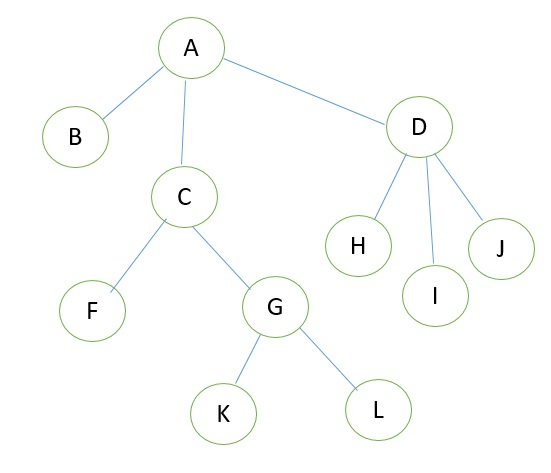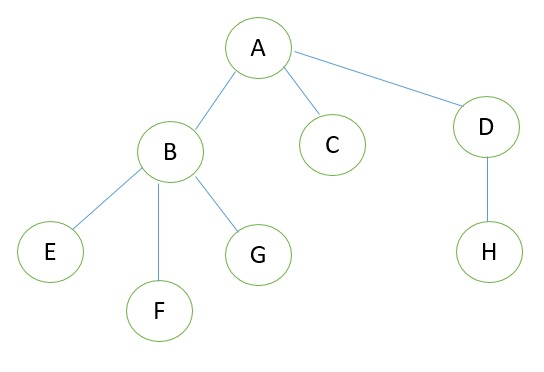# Introduction to trees and its terminologies

Learn: In this article we are going to study about introduction of trees. What is generalized tree? What are different terminologies of tree? Why we use trees? What are benefits of use of trees?
Submitted by Amit Shukla, on October 04, 2017

## Tree

A tree is a collection of elements called nodes. Each node contains some value or element. We will use the term node, rather than vertex with binary tree. Node is the main component of any tree structure. It stores the actual data along with links to other nodes.

### Key properties of Tree

A tree T is represented by nodes and edges, which includes:

1. T is empty (called null or empty tree).
2. T has a left subtree and right subtree.

Example### Tree Terminologies

Consider the following tree1. Root

This is the unique node in the tree in which further subtrees were attached. A root node of a tree has its child. Left child and right child are the two childes a root node can have in a tree. In the given figure A is the root node.

2. Degree of Node

The total number of subtree attached to that node is called the degree of the node. For node A the degree is 3, for node E the degree is 0.

3. Leaf Nodes

These are the terminals nodes of the tree. The nodes which have degree 0 are called as leaf nodes of the tree. These nodes are always present at the end of the tree. Here in our example E, F, C, G, H are the leaf nodes of the tree.

4. Internal Nodes

The nodes in the tree which are other than leaf nodes and the root node are called as internal nodes. These nodes are present in between root node and leaf nodes in the tree that’s why these nodes are called as internal node. Here, B and D are internal nodes.

5. Parent Node

The node which is having further sub branches is called the parent node of those sub branches. In the above figure node B is the parent node of E, F, and G node and E, F, and G are called children of B.

6. Predecessor

While displaying the tree, if some particular nodes previous to some other nodes than that node is called the predecessor of the other node. In our example, the node E is predecessor of node B.

7. Successor

The node which occurs next to some other node is called successor of that node. In our example, B is successor of F and G.

8. Level of tree

The root node is always considering at level zero, and then its adjacent children are supposed to be at level 1 and so on. To understand the concept of level, see the above figure, the node A is at level 0, the node B, C, D are at level 1, the nodes E, F, G, H are at level 2.

9. Height of the tree

The maximum level is the height of the tree. Here the height of the tree is 3. The other terminology used for the height of the tree is depth of the tree.

10. Forest

A tree may be defined as a forest in which only a single node (root) has no predecessor Any forest is consist of collection of trees.

11. Degree of tree

The maximum degree of the node in the tree is called the degree of the tree. Here, the degree of tree is 3.

Top MCQs